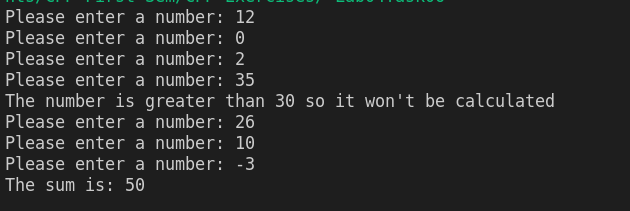# cpp programming exercise with solution

Write a C++ program that should continuously ask the user to input any integer number until and unless a negative number is entered. When a negative number is entered, then the program should end and print the sum of all the entered numbers.

However, it should also check that large numbers (greater than 30) should not be considered for calculating the sum, like shown below:cpp programming exercise with solution

#include <iostream>
using namespace std;

int main()
{
int sum = 0;
int i;
do
{
cin>>i;
if (i>30)
{
cout<<"The number is greater than 30 so it won't be calculated\n";
}
else if (i >= 0)
{
sum += i;
}
i++;
} while (i > 0);
cout<<"The sum is: "<<sum;

return 0;
}